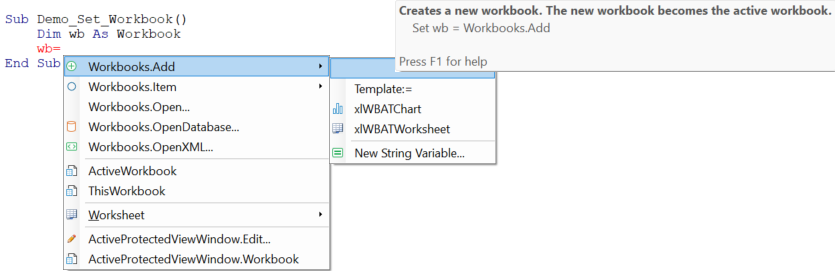﻿ Multiple ways to Set a Workbook

# Set Workbook variable

The correct way to program VBA is to explicitly assign which object you want to work with. In case of a Workbook this can be expressed in several ways which are discussed here. For example, if you want to copy a worksheet, first you will have to tell which worksheet. let's assume `ActiveSheet`, which then begs the question in which workbook it is located.

``````Dim wb As Workbook: Set wb =
Dim ws As Worksheet: Set ws = wb.ActiveSheet
ws.Copy``````There are three groups of methods that can be used to set a Workbook:

If the macro and data are in the same excel file you can use ThisWorkbook. If not, you have more options: the workbook you just opened, the ActiveWorkbook, ...

### ThisWorkbook

If your macro is only intended to work on the excel file that contains it, you would insert `ThisWorkbook`:

``Dim wb As Workbook: Set wb = ThisWorkbook``

### ActiveWorkbook

The Active Workbook is the workbook in the active window (the window on top).

``Dim wb As Workbook: Set wb = ActiveWorkbook``
Notes:
• ActiveWorkbook can also be used in code in the template from which the document will be created. Putting code inside a template requires the template to be available on machines where the workbook is opened.
• ActiveWorkbook can sometimes returns Nothing although in modern Excel versions it seems to be able to assign an open Workbook - if available! It is good practice to test if a workbook was actually set for all methods (except ThisWorkbook).
``````Dim wb As Workbook: Set wb = ...
If wb Is Nothing Then
MsgBox Prompt:="The Workbook is not available", Buttons:=vbOKOnly + vbInformation
Exit Sub
End If``````

### Item in the Workbooks collection

You can specify which workbook using the name or index number. The index count starts with 1, giving the first workbook in the collection.. You can leave the word Item out because the VBA compiler in case of collections uses the Item by default.

``````Dim wb As Workbook
Set wb = Workbooks.Item(1)``````

The name of the active workbook appears in the Excel title bar. If a workbook is new and not yet saved, it will be assigned a temporary name.``Set wb = Workbooks("Book1")``

The Name of the workbook will be filename plus extension in case of a saved workbook, e.g.

``Set wb = Workbooks("Book1.xslx")``

Iterating the collection returns in each round a new workbook variable implicitly set.

``````Dim wb As Workbook
For Each wb In Workbooks

Next wb``````

### Create new or open existing workbook

In many cases the workbook you want to act on is the workbook you just created``````Dim wb As Workbook
``````Dim strFilename As String: strFilename = "C:\temp\book24.xslx"